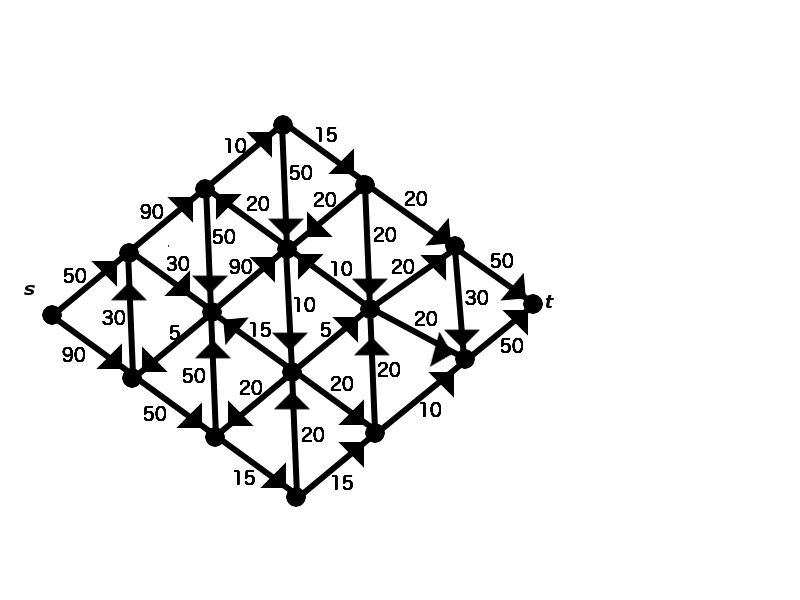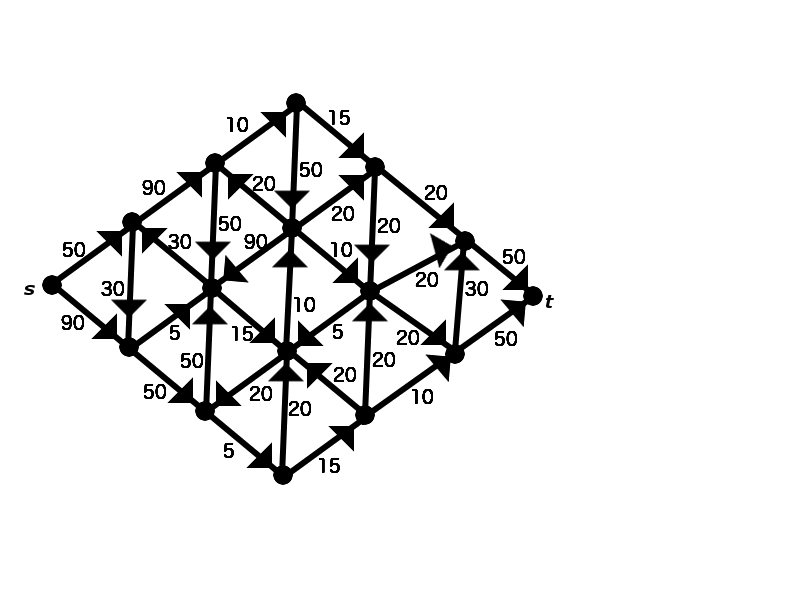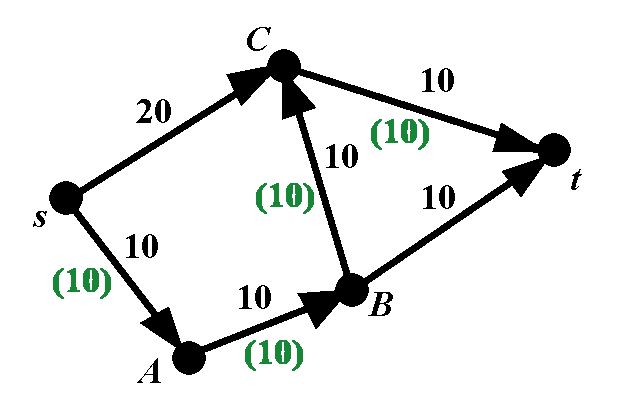MTH4140 Home

# Network Flows and Ford-Fulkerson Algorithm

## Practice problems

Problem 1

Determine the value of the maximal flow from $$s$$ to $$t$$ in the directed graph shown below.Problem 2

Determine the value of the maximal flow from $$s$$ to $$t$$ in the directed graph shown below.Problem 3

The capacities of the edges of a direct graph are given in the picture below. The values of the flow are given by green numbers in brackets. Find an augmenting path for the given flow.